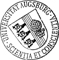## A general spectral approach to the time-domain evolution of linear water waves impacting on a vertical elastic plate

• We present a solution in the time-domain to the two-dimensional linear water-wave problem, in which a semi-infinite fluid region is bounded on one side by a vertical elastic plate. The problem is solved using a generalized eigenfunction expansion from the solutions for single frequencies and we begin with a novel solution of the single-frequency problem. By formulating the problem using the acceleration potential, we find an inner-product space, in which the evolution operator with continuous spectrum is self-adjoint. This inner-product space is required for the generalized eigenfunction solution, which allows to prescribe arbitrary initial water surface and plate displacements and velocities. Furthermore, using the generalized eigenfunction expansion, the solution is approximated by deforming the contour of integration and using the contributions from the singularities of the analytic continuation. Numerical experiments show that the long-time behavior in certain situations can beWe present a solution in the time-domain to the two-dimensional linear water-wave problem, in which a semi-infinite fluid region is bounded on one side by a vertical elastic plate. The problem is solved using a generalized eigenfunction expansion from the solutions for single frequencies and we begin with a novel solution of the single-frequency problem. By formulating the problem using the acceleration potential, we find an inner-product space, in which the evolution operator with continuous spectrum is self-adjoint. This inner-product space is required for the generalized eigenfunction solution, which allows to prescribe arbitrary initial water surface and plate displacements and velocities. Furthermore, using the generalized eigenfunction expansion, the solution is approximated by deforming the contour of integration and using the contributions from the singularities of the analytic continuation. Numerical experiments show that the long-time behavior in certain situations can be well approximated by this method.• Dokument_1.pdfAuthor: Malte A. PeterORCiDGND, Michael H. Meylan urn:nbn:de:bvb:384-opus4-11007 https://opus.bibliothek.uni-augsburg.de/opus4/1307 Preprints des Instituts für Mathematik der Universität Augsburg (2009-24) Preprint English Universität Augsburg Department of Mathematics, University of Auckland 2009/09/24 water waves; spectral theory; generalized eigenfunction expansion; scattering; complex scattering frequency Wasserwelle; Hydroelastizität; Spektraltheorie; Streutheorie Mathematisch-Naturwissenschaftlich-Technische Fakultät Mathematisch-Naturwissenschaftlich-Technische Fakultät / Institut für Mathematik Mathematisch-Naturwissenschaftlich-Technische Fakultät / Institut für Mathematik / Lehr- und Forschungseinheit Angewandte Analysis 5 Naturwissenschaften und Mathematik / 51 Mathematik / 510 MathematikDeutsches Urheberrecht mit Print on Demand